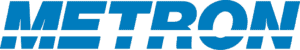## Tracking and Fusion from Multiple Points of View

Depending on your perspective, tracking and fusion can be considered statistical inference problems, probability estimates, or stochastic mathematical models. There is great power in this variety.

### Probabilistic Approach​

Fusion and tracking can also be thought of in probability terms. The desired output is a probability density function (PDF) on the state of the target at a given time. The more spread out the probability density is, the greater is the uncertainty about the target. There are several sources of uncertainty. These include “measurement errors” due to measurements being imperfect (biased and/or imprecise) and “model or process errors” due to the model of the target motion being imperfect. Over time, uncertainty will grow due to the unknown motion of the target. However, as new measurements are received the uncertainty will drop due to the information content in those measurements. Measurements which provide a great deal of new information about the target will cause significant drops in the uncertainty while those which merely confirm what we already know will have a much more limited impact.

### Stochastic Mathematical Approach​

From a mathematical point of view, Tracking is a technique for solving a continuous or discrete vector-valued stochastic differential equation (SDE) where the probability density satisfies Kushner’s equation and probability evolves like heat with sources and sinks subject to convection and diffusion flows.

By considering Fusion and Tracking from these multiple points of view, new innovative techniques can be discovered and applied to problems from many different areas.